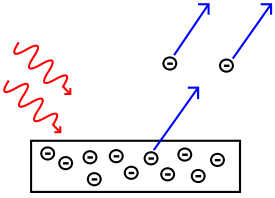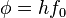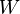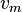# Photoelectric effect

 Photoelectric effectA diagram illustrating the emission of electrons from a metal plate, requiring energy gained from an incoming photon to be more than the work function of the material. Low energy phenomena Photoelectric effect Mid-energy phenomena Compton scattering High energy phenomena Pair production

The photoelectric effect is a quantum electronic phenomenon in which electrons are emitted from matter after the absorption of energy from electromagnetic radiation such as x-rays or visible light. The emitted electrons can be referred to as photoelectrons in this context. The effect is also termed the Hertz Effect, based on its discovery by Heinrich Hertz, although the term has generally fallen out of use.

Study of the photoelectric effect led to important steps in understanding the quantum nature of light and electrons and influenced the formation of the concept of wave–particle duality.

The term may also refer to the photoconductive effect (also known as photoconductivity or photoresistivitity), the photovoltaic effect, or the photoelectrochemical effect.

## Introduction

When a metallic surface is exposed to electromagnetic radiation above a certain threshold frequency, the light is absorbed and electrons are emitted. In 1902, Philipp Eduard Anton von Lenard observed that the energy of the emitted electrons increased with the frequency, or color, of the light. This was at odds with James Clerk Maxwell's wave theory of light, which predicted that the energy would be proportional to the intensity of the radiation. In 1905, Albert Einstein solved this paradox by describing light as composed of discrete quanta, now called photons, rather than continuous waves. Based upon Max Planck's theory of black-body radiation, Einstein theorized that the energy in each quantum of light was equal to the frequency multiplied by a constant, later called Planck's constant. A photon above a threshold frequency has the required energy to eject a single electron, creating the observed effect. This discovery led to the quantum revolution in physics and earned Einstein the Nobel Prize in 1921.

## Explanation

The photons of the light beam have a characteristic energy determined by the frequency of the light. In the photoemission process, if an electron absorbs the energy of one photon and has more energy than the work function (the electron binding energy), it is ejected from the material. If the photon energy is too low, the electron is unable to escape the surface of the material. Increasing the intensity of the light beam increases the number of photons in the light beam, and thus increases the number of electrons emitted without increasing the energy that each electron possesses. Thus the energy of the emitted electrons does not depend on the intensity of the incoming light, but only on the energy of the individual photons.

Electrons can absorb energy from photons when irradiated, but they follow an "all or nothing" principle. All of the energy from one photon must be absorbed and used to liberate one electron from atomic binding, or the energy is re-emitted. If the photon energy is absorbed, some of the energy liberates the electron from the atom, and the rest contributes to the electron's kinetic energy as a free particle.

### Experimental results of the photoelectric emission

1. For a given metal and frequency of incident radiation, the rate at which photoelectrons are ejected is directly proportional to the intensity of the incident light.
2. For a given metal, there exists a certain minimum frequency of incident radiation below which no photoelectrons can be emitted. This frequency is called the threshold frequency.
3. Above the threshold frequency, the maximum kinetic energy of the emitted photoelectron is independent of the intensity of the incident light but depends on the frequency of the incident light.
4. The time lag between the incidence of radiation and the emission of a photoelectron is very small, less than 10-9 second.

### Equations

In effect quantitatively using Einstein's method, the following equivalent equations are used:

Energy of photon = Energy needed to remove an electron + Kinetic energy of the emitted electron

Algebraically:$hf = \phi + E_{k_{max}} \,$

where

• h is Planck's constant,
• f is the frequency of the incident photon,
•$\phi = h f_0 \$ is the work function (sometimes denoted$W$ instead), the minimum energy required to remove a delocalized electron from the surface of any given metal,
•$E_{k_{max}} = \frac{1}{2} m v_m^2$ is the maximum kinetic energy of ejected electrons,
• f0 is the threshold frequency for the photoelectric effect to occur,
• m is the rest mass of the ejected electron, and
•$v_m$ is the speed of the ejected electron.

Since an emitted electron cannot have negative kinetic energy, the equation implies that if the photon's energy (hf) is less than the work function ($\phi$), no electron will be emitted.

According to Einstein's special theory of relativity the relation between energy (E) and momentum (p) of a particle is$E = \sqrt{(pc)^2 + (mc^2)^2}$, where m is the rest mass of the particle and c is the velocity of light in a vacuum.

### Three-step model

The photoelectric effect in crystalline material is often decomposed into three steps:

1. Inner photoelectric effect (see photodiode below). The hole left behind can give rise to auger effect, which is visible even when the electron does not leave the material. In molecular solids photons are excited in this step and may be visible as lines in the final electron energy. The inner photoeffect has to be dipole allowed. The transition rules for atoms translate via the tight-binding model onto the crystal. They are similar in geometry to plasma oscillations in that they have to be transversal.
2. Ballistic transport of half of the electrons to the surface. Some electrons are scattered.
3. Electrons escape from the material at the surface.

In the three-step model, an electron can take multiple paths through these three steps. All paths can interfere in the sense of the path integral formulation. For surface states and molecules the three-step model does still make some sense as even most atoms have multiple electrons which can scatter the one electron leaving.

## History

### Early observations

In 1839, Alexandre Edmond Becquerel observed the photoelectric effect via an electrode in a conductive solution exposed to light. In 1873, Willoughby Smith found that selenium is photoconductive.

### Hertz's spark gaps

In 1887, a young German scientist named Heinrich Hertz observed the photoelectric effect and the production and reception of electromagnetic (EM) waves. He published these observations in the journal Annalen der Physik. His receiver consisted of a coil with a spark gap, where a spark would be seen upon detection of EM waves. He placed the apparatus in a darkened box to see the spark better. However, he noticed that the maximum spark length was reduced when in the box. A glass panel placed between the source of EM waves and the receiver absorbed ultraviolet radiation that assisted the electrons in jumping across the gap. When removed, the spark length would increase. He observed no decrease in spark length when he substituted quartz for glass, as quartz does not absorb UV radiation. Hertz concluded his months of investigation and reported the results obtained. He did not further pursue investigation of this effect, nor did he make any attempt at explaining how this phenomenon was brought about.

### J.J. Thomson: electrons

In 1899, J. J. Thomson investigated ultraviolet light in Crookes tubes. Influenced by the work of James Clerk Maxwell, Thomson deduced that cathode rays consisted of negatively charged particles, later called electrons, which he called "corpuscles." In the research, Thomson enclosed a metal plate (a cathode) in a vacuum tube, and exposed it to high frequency radiation. It was thought that the oscillating electromagnetic fields caused the atoms' field to resonate and, after reaching a certain amplitude, caused a subatomic "corpuscle" to be emitted, and current to be detected. The amount of this current varied with the intensity and color of the radiation. Larger radiation intensity or frequency would produce more current.

Nikola Tesla described the photoelectric effect in 1901. He described such radiation as vibrations of aether of small wavelengths which ionized the atmosphere. On November 5, 1901, he received the patent US685957 (Apparatus for the Utilization of Radiant Energy) that describes radiation charging and discharging conductors (e.g., a metal plate or piece of mica) by "radiant energy." Tesla used this effect to charge a capacitor with energy by means of a conductive plate (i.e., a solar cell precursor). The radiant energy threw off with great velocity minute particles (i.e., electrons) which were strongly electrified. The patent specified that the radiation (or radiant energy) included many different forms. These devices have been referred to as "Photoelectric alternating current stepping motors."

In practice, a polished metal plate in radiant energy (e.g. sunlight) will gain a positive charge as electrons are emitted by the plate. As the plate charges positively, electrons form an electrostatic force on the plate (because of surface emissions of the photoelectrons), and "drain" any negatively charged capacitors. As the rays or radiation fall on the insulated conductor (which is connected to a capacitor), the condenser will indefinitely charge electrically.

### Von Lenard's observations

In 1902, Philipp Eduard Anton von Lenard observed the variation in electron energy with light frequency. He used a powerful electric arc lamp which enabled him to investigate large changes in intensity, and had sufficient power to enable him to investigate the variation of potential with light frequency. His experiment directly measured potentials, not electron kinetic energy: he found the electron energy by relating it to the maximum stopping potential (voltage) in a phototube. He found that the calculated maximum electron kinetic energy is determined by the frequency of the light. For example, an increase in frequency results in an increase in the maximum kinetic energy calculated for an electron upon liberation - ultraviolet radiation would require a higher applied stopping potential to stop current in a phototube than blue light. However Lenard's results were qualitative rather than quantitative because of the difficulty in performing the experiments: the experiments needed to be done on freshly cut metal so that the pure metal was observed, but it oxidized in a matter of minutes even in the partial vacuums he used. The current emitted by the surface was determined by the light's intensity, or brightness: doubling the intensity of the light doubled the number of electrons emitted from the surface. Lenard did not know of photons.

### Einstein: light quanta

Albert Einstein's mathematical description in 1905 of how the photoelectric effect was caused by absorption of quanta of light (now called photons), was in the paper named "On a Heuristic Viewpoint Concerning the Production and Transformation of Light." This paper proposed the simple description of "light quanta," or photons, and showed how they explained such phenomena as the photoelectric effect. His simple explanation in terms of absorption of single quanta of light explained the features of the phenomenon and the characteristic frequency. Einstein's explanation of the photoelectric effect won him the Nobel Prize in Physics in 1921.

The idea of light quanta began with Max Planck's published law of black-body radiation ("On the Law of Distribution of Energy in the Normal Spectrum." Annalen der Physik 4 (1901)) by assuming that Hertzian oscillators could only exist at energies E proportional to the frequency f of the oscillator by E = hf, where h is Planck's constant. By assuming that light actually consisted of discrete energy packets, Einstein wrote an equation for the photoelectric effect that fit experiments (it explained why the energy of the photoelectrons was dependent only on the frequency of the incident light and not on its intensity: a low intensity, high frequency source could supply a few high energy photons, whereas a high intensity, low frequency source would supply no photons of sufficient individual energy to dislodge any electrons). This was an enormous theoretical leap, and the reality of the light quanta was strongly resisted. The idea of light quanta contradicted the wave theory of light that followed naturally from James Clerk Maxwell's equations for electromagnetic behavior and more generally, the assumption of infinite divisibility of energy in physical systems. Even after experiments showed that Einstein's equations for the photoelectric effect were accurate, resistance to the idea of photons continued, since it appeared to contradict Maxwell's equations, which were well understood and verified.

Einstein's work predicted that the energy of the ejected electrons increases linearly with the frequency of the light. Perhaps surprisingly, that had not yet been tested. In 1905 it was known that the energy of the photoelectrons increased with increasing frequency of incident light – and independent of the intensity of the light. However, the manner of the increase was not experimentally determined to be linear until 1915 when Robert Andrews Millikan showed that Einstein was correct.

### Effect on wave–particle question

The photoelectric effect helped propel the then-emerging concept of the dualistic nature of light, that light exhibits characteristics of waves and particles at different times. The effect was impossible to understand in terms of the classical wave description of light, as the energy of the emitted electrons did not depend on the intensity of the incident radiation. Classical theory predicted that the electrons could 'gather up' energy over a period of time, and then be emitted. For such a classical theory to work a pre-loaded state would need to persist in matter. The idea of the pre-loaded state was discussed in Millikan's book Electrons (+ & –) and in Compton and Allison's book X-Rays in Theory and Experiment.

## Uses and Effects

### Photodiodes and phototransistors

Solar cells (used in solar power) and light-sensitive diodes use a variant of the photoelectric effect, but not ejecting electrons out of the material. In semiconductors, light of even relatively low energy, such as visible photons, can kick electrons out of the valence band and into the higher-energy conduction band, where they can be harnessed, creating electric current at a voltage related to the bandgap energy.

### Image sensors

Video camera tubes in the early days of television used the photoelectric effect; newer variants used photoconductive rather than photoemissive materials.

Silicon image sensors, such as charge-coupled devices, widely used for photographic imaging, are based on a variant of the photoelectric effect, in which photons knock electrons out of the valence band of energy states in a semiconductor, but not out of the solid itself.

### The gold-leaf electroscope

Gold-leaf electroscopes are designed to detect static electricity. Charge placed on the metal cap spreads to the stem and the gold leaf of the electroscope. Because they then have the same charge, the stem and leaf repel each other. This will cause the leaf to bend away from the stem.

The electroscope is an important tool in illustrating the photoelectric effect. Let us say that the scope is negatively charged throughout. There is an excess of electrons and the leaf is separated from the stem. But if we then shine high-frequency light onto the cap, the scope discharges and the leaf will fall limp. This is because the frequency of the light shining on the cap is above the cap's threshold frequency. The photons in the light have enough energy to liberate electrons from the cap, reducing its negative charge. This will discharge a negatively charged electroscope and further charge a positive electroscope.

However, if the EM radiation hitting the metal cap does not have a high enough frequency, (its frequency is below the threshold value for the cap) then the leaf will never discharge, no matter how long one shines the low-frequency light at the cap.

### Photoelectron spectroscopy

Since the energy of the photoelectrons emitted is exactly the energy of the incident photon minus the material's work function or binding energy, the work function of a sample can be determined by bombarding it with a monochromatic X-ray source or UV source (typically a helium discharge lamp), and measuring the kinetic energy distribution of the electrons emitted.

Photoelectron spectroscopy is done in a high vacuum environment, since the electrons would be scattered by air.

A typical electron energy analyzer is a concentric hemispherical analyzer (CHA), which uses an electric field to divert electrons different amounts depending on their kinetic energies. For every element and core (atomic orbital) there will be a different binding energy. The many electrons created from each will then show up as spikes in the analyzer, and can be used to determine the elemental composition of the sample.

### Spacecraft

The photoelectric effect will cause spacecraft exposed to sunlight to develop a positive charge. This can get up to the tens of volts. This can be a major problem, as other parts of the spacecraft in shadow develop a negative charge (up to several kilovolts) from nearby plasma, and the imbalance can discharge through delicate electrical components. The static charge created by the photoelectric effect is self-limiting, though, because a more highly-charged object gives up its electrons less easily.

### Moon dust

Light from the sun hitting lunar dust causes it to become charged through the photoelectric effect. The charged dust then repels itself and lifts off the surface of the Moon by electrostatic levitation. This manifests itself almost like an "atmosphere of dust," visible as a thin haze and blurring of distant features, and visible as a dim glow after the sun has set. This was first photographed by the Surveyor program probes in the 1960s. It is thought that the smallest particles are repelled up to kilometers high, and that the particles move in "fountains" as they charge and discharge.

### Night vision devices

Photons hitting a gallium arsenide plate in night vision devices cause the ejection of photoelectrons due to the photoelectric effect. These are then amplified into a cascade of electrons that light up a phosphor screen.